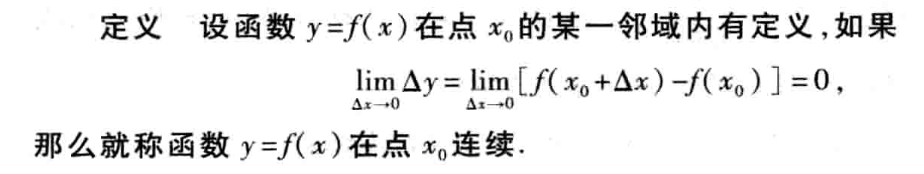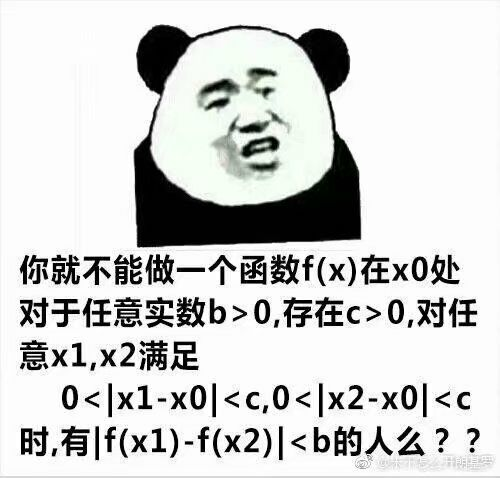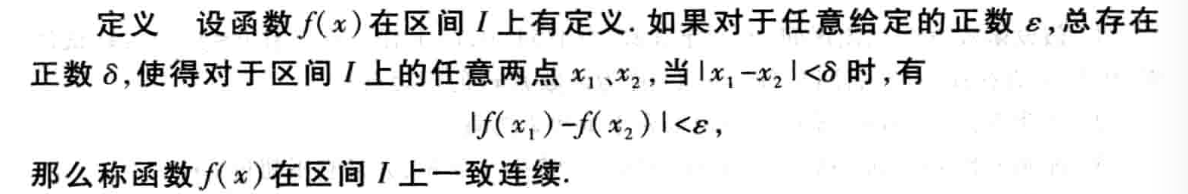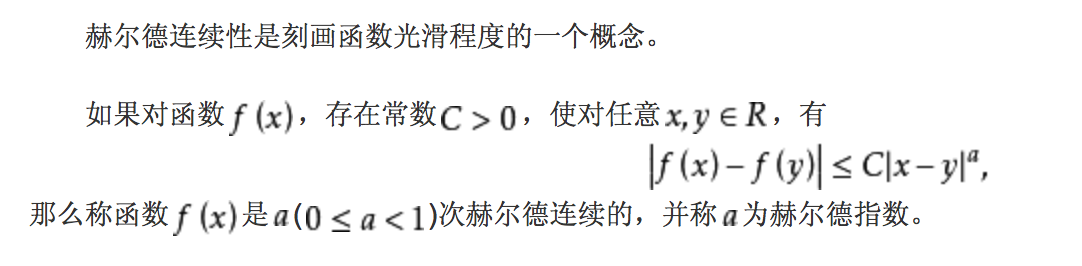• ## 一致连续

千次阅读 2014-03-05 17:36:10
根据定义：设函数f(x)在区间I上有定义，如果对于任意给定的正数a,总存在着正数b,使得对于区间I上的任意两点x1,x2,当x1-x2的绝对值小于b时，就有f(x1)-f(x2)的绝对值小于a,那么就称函数f(x)在区间I上是一致连续的。...


根据定义：设函数f(x)在区间I上有定义，如果对于任意给定的正数a,总存在着正数b,使得对于区间I上的任意两点x1,x2,当x1-x2的绝对值小于b时，就有f(x1)-f(x2)的绝对值小于a,那么就称函数f(x)在区间I上是一致连续的。

一致连续要看定义域的选择，而且与其有紧密联系，一致连续的几何意义：其实很简单，就是函数值不能变化太大，从图像上来看就是不能垂直x轴。

一致连续是一个整体性概念，与函数的有界性、可积性类似，说一个函数一致连续必须提到在某个区间上一致连续；而连续性则是一个局部性概念，与函数在一点有极限类似，可以说函数在某一点连续，但不能说函数在某一点一致连续。在这种意义下提几何意义只能笼统地讲，函数图象变化的不是非常剧烈。实际上一致连续性一般用于函数的整体性质的证明，例如连续函数的可积性证明等。

连续是逐点定义的，连续函数在定义区间上点点都连续的函数，一致连续是更强的连续，不仅连续而且连续变化不能太快，
几何意义：在所定义的区间上应该有最陡峭的位置，也即变化最快的位置，并且这个变化最快的程度有限的。
例如，反比例函数1/x.在区间（0，1）没有最陡峭的位置，[0.00000001，80000）就有最陡峭的位置0.00000001点


展开全文• ## 二元函数的连续性

千次阅读 2019-09-28 19:37:32
文章目录二元连续的定义增量复合函数的连续性有界闭域上连续函数的性质有界性and最大最小值定理证明一致连续性证明介值性定理证明 二元连续的定义 fff是定义在点集D⊂R2D\subset R^2D⊂R2上的二元 P0∈D(它是D...
文章目录二元连续的定义增量复合函数的连续性有界闭域上连续函数的性质有界性and最大最小值定理证明一致连续性证明介值性定理证明
二元连续的定义

$f$是定义在点集$D\subset R^2$上的二元
$P_0\in D(它是D的聚点或孤立点)$
对于$\forall\varepsilon>0,总\exist\delta>0,只要P\in U(P_0;\delta)\cap D,$就有$|f(P)-f(P_0)|<\varepsilon$则称$f$关于集合D在点$P_0$连续

注意~

若$P_0$是孤立点，则$P_0$肯定是$f$的连续点，因为$U(P_0;\delta)\cap D$只有$P_0$点
若是聚点，则以上定义等价于$\lim\limits_{P\to P_0,P\in D}f(P)=f(P_0)$
若$P_0$是聚点，而上式不成立，则称$P_0$是$f$的不连续点或间断点；
4.若极限存在只是$\ne f(P_0)$,则称该点为可去间断点

增量

设$P_0(x_0,y_0),P(x,y)\in D$ $\triangle x=x-x_0,\triangle y=y-y_0,$
全增量：$\triangle z=\triangle f(x_0,y_0)$ $=f(x,y)-f(x_0,y_0)$ $=f(x_0+\triangle x,y_0+\triangle y)-f(x_0,y_0)$以上都称为$f$在点$P_0$的全增量

用增量定义连续：当$\lim\limits_{(\triangle x,\triangle y)\to(0,0),(x,y)\in D}\triangle z=0$

偏增量：在全增量中令$\triangle x=0$或$\triangle y=0$,即$\triangle_xf(x_0,y_0)=f(x_0+\triangle x,y_0)-f(x_0,y_0)$ $\triangle_yf(x_0,y_0)=f(x_0,y_0+\triangle y)-f(x_0,y_0)$

若偏增量的极限为0，如$\lim\limits_{\triangle x\to0}\triangle_xf(x_0,y_0)=0$表示固定$y=y_0$时，$f(x,y_0)$作为x的一元函数在$x_0$处连续
而$f(x,y)$在内点$(x_0,y_0)$处连续，可以推出$f(x,y_0)$在$x_0$处连续，$f(x_0,y)$在$y_0$处连续

复合函数的连续性

$u=\varphi(x,y),v=\psi(x,y)$在$xy$平面上的点$P_0(x_0,y_0)$某邻域有定义且在该点连续
$f(u,v)$在$uv$平面上的点$Q_0(u_0,v_0)$某领域有定义，也在$Q_0$连续
$u_0=\varphi(x_0,y_0),v_0=\psi(x_0,y_0)$
则，$g(x,y)=f[\varphi(x,y),\psi(x,y)]$在$P_0$处也连续

$f(u,v)$在$Q_0$连续可以描述为：
对$\forall\varepsilon>0,\exist\eta>0$，使得当$|u-u_0|<\eta,|v-v_0|<\eta$时，有$|f(u,v)-f(u_0,v_0)|<\varepsilon$
有界闭域上连续函数的性质
有界性and最大最小值定理
$f$在有界闭域$D\subset R^2$连续，则

$f$在D上有界
且能取到最大最小值

证明

先证明$f$的有界性：

反证，假设无界，则对所有正整数$n$，必$\exist P_n\in D$，使得 $|f(P_n)|>n,n=1,2,...\tag{1}$
于是得到一有界点列$\{P_n\}\subset D$,且该点列有无穷多个点
由有界无限点列必存在收敛的子列得，$\{P_n\}$存在收敛子列$\{P_{n_k}\}$，设$\lim\limits_{k\to\infty}P_{n_k}=P_0$由于D是闭域，因此$P_0\in D$(这说明，若D是开域，则有可能收敛到界点)
由于$f$在D上连续，所以有$\lim\limits_{k\to\infty}f(P_{n_k})=f(P_0)$这与不等式(1)矛盾，所以$f$在D上有界

下证$f$能取到最大最小值：

设$m=\mathop{inf}f(D),M=supf(D)$这里证明必存在一点$Q\in D$，使得$f(Q)=M$最小值类似
依然反证，假设不然，则对$\forall P\in D$，都有$M-f(P)>0$构造一正值函数$F(P)=\frac 1{M-f(P)}$由于F在D上也连续，由以上证明可知，F在D上有界
又∵$f$在D上不能达到上确界$M$，所以存在收敛点列$\{P_n\}\subset D,$使得$\lim\limits_{n\to\infty}f(P_n)=M$这样的话就有$\lim\limits_{n\to\infty}F(P)=+\infty$与F有界的结论矛盾了，所以证得$f$在D上可以取到最大值

一致连续性
$f$在有界闭域$D\subset R^2$连续，则

$f$在D上一致连续
即，对$\forall\varepsilon>0,\exist \delta(\varepsilon)>0,对\forall P,Q,$只要满足$\rho(P,Q)<\delta$，就有$|f(P)-f(Q)|<\varepsilon$

证明

用聚点定理
套话系列：若$f$在D上连续却不一致连续，则

$\exist\varepsilon_0>0,$对于任意小的$\delta>0$，比如$\delta=\frac 1n,n=1,2,...,$，总有相应的$P_n,Q_n\in D$
即使$\rho(P_n,Q_n)<\frac 1n$
但是$|f(P_n)-f(Q_n)|\ge \varepsilon_0$

由于$D$为有界闭域，故存在收敛子列$\{P_{n_k}\}\in \{P_n\}$,设$P_{n_k}\to P_0\in D(k\to\infty)$
方便起见，在$\{Q_n\}$中取出与$P_{n_k}$相同的子列$\{Q_{n_k}\}$，则有$0\le\rho(P_{n_k},Q_{n_k})<\frac 1{n_k}\to0,k\to\infty$
所以有$\lim\limits_{k\to\infty}Q_{n_k}=\lim\limits_{k\to\infty}P_{n_k}=P_0$
又∵$f$在$P_0$处连续，所以有$\lim\limits_{k\to\infty}|f(P_{n_k})-f(Q_{n_k})|$ $=|f(P_0)-f(P_0)|=0$
与$|f(P_n)-f(Q_n)|\ge \varepsilon_0>0$矛盾，所以$f$在D上一致连续

介值性定理
$f$在区域$D\subset R^2$连续

$P_1,P_2$为D上任意两点
$f(P_1)
若$\mu$满足$f(P_1)<\mu
则必存在一点$P_0\in D,使得$ $f(P_0)=\mu$

证明

注意，这里一定要是区域，因为要用到区域的连通性质,而有界性定理和一致连续定理其实条件都可以改成有界闭集
做辅助函数$F(P)=f(P)-\mu$可得：F在D上连续，且有$F(P_1)<0,F(P_2)>0$
不妨设$P_1,P_2$是D的内点，下面证明必存在$P_0\in D,$使得$F(P_0)=\mu$

由于D为区域，所以肯定有一段有限折线连接$P_1,P_2$，若有一连接点的函数值=0.则定理可证，否则从一端开始逐段检查线段，必存在某段，F在两端函数值异号
设连接$P_1(x_1,y_1),P_2(x_2,y_2)$的直线段含于D，方程为$\begin{cases}x=x_1+t(x_2-x_1)\\y=y_1+t(y_2-y_1)\end{cases},0\le t\le1$
在该直线段上，F表示为关于t的复合$G(t)=F(x_1+t(x_2-x_1),y_1+t(y_2-y_1))$ $0\le t\le1$啊！这就构造好了一个$[0,1]$上的一元连续函数啦,可以用介值定理了！$F(P_1)=G(0)<0根的存在性定理，在(0,1)内存在一点$t_0$,使得$G(t_0)=0$,记$x_0=x_1+t_0(x_2-x_1)$ $y_0=y_1+t_0(y_2-y_1)$就有$P_0\in D$使得$F(P_0)=G(t_0)=0,f(P_0)=\mu$


展开全文• 函数的连续性与间断点 函数的连续性 函数的间断点 ...一致连续性函数的连续性与间断点函数的连续性 设函数y=f(x)y=f(x)在点x0x_0的某一邻域内有定义，如果limΔx→0Δy=limΔx→0[f(x0+Δx)−f(x0)


函数的连续性与间断点
函数的连续性
函数的间断点

连续函数的运算与初等函数的连续性
连续函数的和、差、积、商的连续性
反函数与复合函数的连续性
初等函数的连续性

闭区间上连续函数的性质
有界性与最大值最小值定理
零点定理与介值定理
一致连续性

函数的连续性与间断点

函数的连续性

设函数y=f(x)$y=f\left(x\right)$$y=f(x)$在点x0${x}_{0}$$x_0$的某一邻域内有定义，如果limΔx→0Δy=limΔx→0[f(x0+Δx)−f(x0)]=0$\underset{\mathrm{\Delta }x\to 0}{lim}\mathrm{\Delta }y=\underset{\mathrm{\Delta }x\to 0}{lim}\left[f\left({x}_{0}+\mathrm{\Delta }x\right)-f\left({x}_{0}\right)\right]=0$$\lim_{\Delta x\rightarrow 0}\Delta y = \lim_{\Delta x \rightarrow 0}[f(x_0+\Delta x) - f(x_0)] = 0$，那么就称函数y=f(x)$y=f\left(x\right)$$y=f(x)$在点x0${x}_{0}$$x_0$连续
设函数y=f(x)$y=f\left(x\right)$$y=f(x)$在点x0${x}_{0}$$x_0$的某一邻域内有定义，如果limx→x0f(x)=f(x0)$\underset{x\to {x}_{0}}{lim}f\left({x}_{\right)}=f\left({x}_{0}\right)$$\lim_{x\rightarrow x_0}f(x_)=f(x_0)$，那么就称函数f(x)$f\left(x\right)$$f(x)$在点x0${x}_{0}$$x_0$连续
如果limx→x−0f(x)=f(x−0)$\underset{x\to {x}_{0}^{-}}{lim}f\left(x\right)=f\left({x}_{0}^{-}\right)$$\lim_{x\rightarrow x_0^-}f(x)=f(x_0^-)$存在且等于f(x0)$f\left({x}_{0}\right)$$f(x_0)$，即f(x−0)=f(x0)$f\left({x}_{0}^{-}\right)=f\left({x}_{0}\right)$$f(x_0^-)=f(x_0)$，就说函数f(x)$f\left(x\right)$$f(x)$在点x0${x}_{0}$$x_0$左连续；如果limx→x+0f(x)=f(x+0)$\underset{x\to {x}_{0}^{+}}{lim}f\left(x\right)=f\left({x}_{0}^{+}\right)$$\lim_{x\rightarrow x_0^+}f(x)=f(x_0^+)$存在且等于f(x0)$f\left({x}_{0}\right)$$f(x_0)$，即f(x+0)=f(x0)$f\left({x}_{0}^{+}\right)=f\left({x}_{0}\right)$$f(x_0^+)=f(x_0)$，就说函数f(x)$f\left(x\right)$$f(x)$在点x0${x}_{0}$$x_0$右连续
在区间上每一点都连续的函数，叫做在该区间上连续函数，或者说函数在该区间上连续

函数的间断点

设函数f(x)$f\left(x\right)$$f(x)$在点x0${x}_{0}$$x_0$的某去心邻域内有定义，在此前提下，如果函数f(x)$f\left(x\right)$$f(x)$有下列三种情形之一：
在x=x0$x={x}_{0}$$x=x_0$没有定义；
虽在x=x0$x={x}_{0}$$x=x_0$有定义，但limx→x0f(x)$\underset{x\to {x}_{0}}{lim}f\left(x\right)$$\lim_{x\rightarrow x_0}f(x)$不存在；
虽在x=x0$x={x}_{0}$$x=x_0$有定义，且limx→x0f(x)$\underset{x\to {x}_{0}}{lim}f\left(x\right)$$\lim_{x\rightarrow x_0}f(x)$存在，但limx→x0f(x)≠f(x0)$\underset{x\to {x}_{0}}{lim}f\left(x\right)\ne f\left({x}_{0}\right)$$\lim_{x\rightarrow x_0}f(x) \not= f(x_0)$，
则函数f(x)$f\left(x\right)$$f(x)$在点x0${x}_{0}$$x_0$为不连续，而点x0${x}_{0}$$x_0$称为函数f(x)$f\left(x\right)$$f(x)$的不连续点或间断点。常见间断点：无穷间断点、震荡间断点、可去间断点、跳跃间断点
若间断点的左右极限都存在，那么间断点称为函数f(x)$f\left(x\right)$$f(x)$的第一类间断点，否则称为第二类间断点

连续函数的运算与初等函数的连续性

连续函数的和、差、积、商的连续性

设函数f(x)$f\left(x\right)$$f(x)$和g(x)$g\left(x\right)$$g(x)$在点x0${x}_{0}$$x_0$连续，则它们的和（差）f±g$f±g$$f\pm g$、积f⋅g$f\cdot g$$f\cdot g$及商fg$\frac{f}{g}$$\frac{f}{g}$（当g(x0)≠0$g\left({x}_{0}\right)\ne 0$$g(x_0)\not=0$时）都在点x0${x}_{0}$$x_0$连续

反函数与复合函数的连续性

如果函数y=f(x)$y=f\left(x\right)$$y=f(x)$在区间Ix${I}_{x}$$I_x$上单调增加（或单调减少）且连续，那么它的反函数x=f−1(y)$x={f}^{-1}\left(y\right)$$x=f^{-1}(y)$也在对应的区间Iy={y|y=f(x),x∈Ix}${I}_{y}=\left\{y|y=f\left(x\right),x\in {I}_{x}\right\}$$I_y=\{y|y=f(x),x\in I_x\}$上单调增加（或单调减少）且连续
设函数y=f[g(x)]$y=f\left[g\left(x\right)\right]$$y=f[g(x)]$由函数u=g(x)$u=g\left(x\right)$$u=g(x)$与函数y=f(x)$y=f\left(x\right)$$y=f(x)$复合而成，U˚(x0)⊂Df∘g$\stackrel{˚}{U}\left({x}_{0}\right)\subset {D}_{f\circ g}$$\mathring{U}(x_0)\subset D_{f\circ g}$。若limx→x0g(x)=u0$\underset{x\to {x}_{0}}{lim}g\left(x\right)={u}_{0}$$\lim_{x\rightarrow x_0} g(x)=u_0$，而函数y=f(u)$y=f\left(u\right)$$y=f(u)$在u=u0$u={u}_{0}$$u=u_0$连续，则limx→x0f[g(x)]=limu→u0f(u)=f(u0)$\underset{x\to {x}_{0}}{lim}f\left[g\left(x\right)\right]=\underset{u\to {u}_{0}}{lim}f\left(u\right)=f\left({u}_{0}\right)$$\lim_{x\rightarrow x_0}f[g(x)]=\lim_{u\rightarrow u_0}f(u)=f(u_0)$
设函数y=f[g(x)]$y=f\left[g\left(x\right)\right]$$y=f[g(x)]$是由函数u=g(x)$u=g\left(x\right)$$u=g(x)$与函数y=f(u)$y=f\left(u\right)$$y=f(u)$复合而成，U(x0)⊂Df∘g$U\left({x}_{0}\right)\subset {D}_{f\circ g}$$U(x_0)\subset D_{f\circ g}$。若函数u=g(x)$u=g\left(x\right)$$u=g(x)$在x=x0$x={x}_{0}$$x=x_0$连续，且g(x0)=u0$g\left({x}_{0}\right)={u}_{0}$$g(x_0)=u_0$，而函数y=f(u)$y=f\left(u\right)$$y=f(u)$在u=u0$u={u}_{0}$$u=u_0$连续，则复合函数y=f[g(x)]$y=f\left[g\left(x\right)\right]$$y=f[g(x)]$在x=x0$x={x}_{0}$$x=x_0$也连续

初等函数的连续性

一切初等函数在其定义区间内都是连续的。定义区间就是包含在定义域内的区间

闭区间上连续函数的性质

有界性与最大值最小值定理

对于在区间I$I$$I$上有定义的函数f(x)$f\left(x\right)$$f(x)$，如果有x0∈I${x}_{0}\in I$$x_0\in I$，使得对于任一x∈I$x\in I$$x\in I$都有f(x)≤f(x0)(f(x)≥f(x0))$f\left(x\right)\le f\left({x}_{0}\right)\left(f\left(x\right)\ge f\left({x}_{0}\right)\right)$$f(x) \le f(x_0) (f(x) \ge f(x_0))$，则称f(x0)$f\left({x}_{0}\right)$$f(x_0)$是函数f(x)$f\left(x\right)$$f(x)$在区间I$I$$I$上的最大值（最小值）
有界性与最大值最小值定理：在闭区间上连续的函数在该区间上有界且一定能取得它的最大值和最小值

零点定理与介值定理

零点定理：设函数f(x)$f\left(x\right)$$f(x)$在闭区间[a,b]$\left[a,b\right]$$[a,b]$上连续，且f(a)$f\left(a\right)$$f(a)$与f(b)$f\left(b\right)$$f(b)$异号（即f(a)⋅f(b)<0$f\left(a\right)\cdot f\left(b\right)<0$$f(a)\cdot f(b)<0$），那么在开区间(a,b)$\left(a,b\right)$$(a,b)$内至少有一点ξ$\xi$$\xi$，使得f(ξ)=0$f\left(\xi \right)=0$$f(\xi)=0$
介值定理：设函数f(x)$f\left(x\right)$$f(x)$在闭区间[a,b]$\left[a,b\right]$$[a,b]$上连续，且在这区间的端点取不同的函数值f(a)=A$f\left(a\right)=A$$f(a)=A$及f(b)=B$f\left(b\right)=B$$f(b)=B$，那么，对于A$A$$A$与B$B$$B$之间的任意一个数C$C$$C$，在开区间(a,b)$\left(a,b\right)$$(a,b)$内至少有一点ξ$\xi$$\xi$，使得f(ξ)=C(a<ξ<b)$f\left(\xi \right)=C\left(a<\xi $f(\xi)=C(a<\xi
在闭区间上连续的函数必取得介于最大值M$M$$M$与最小值m$m$$m$之间的任何值

一致连续性

设函数f(x)$f\left(x\right)$$f(x)$在区间I$I$$I$上有定义，如果对于任意给定的正数ϵ$ϵ$$\epsilon$，总存在着正数δ$\delta$$\delta$，使得对于区间I$I$$I$上任意两点x1、x2${x}_{1}、{x}_{2}$$x_1、x_2$，当|x1−x2|<δ$|{x}_{1}-{x}_{2}|<\delta$$|x_1-x_2|<\delta$时，就有|f(x1)−f(x2)|<ϵ$|f\left({x}_{1}\right)-f\left({x}_{2}\right)|<ϵ$$|f(x_1)-f(x_2)|<\epsilon$，那么称函数f(x)$f\left(x\right)$$f(x)$在区间I$I$$I$上是一致连续的
一致连续性定理：如果函数f(x)$f\left(x\right)$$f(x)$在闭区间[a,b]$\left[a,b\right]$$[a,b]$上连续，那么它在该区间上一致连续

展开全文函数 数学
• 个人理解，连续是个局部的概念，一般说函数在某一点x0处，满足那样一个性质，就说这个函数在x0连续。 同济第七版的定义是这个样子的： 表情包版本的定义是这个样子的： 定义的版本各种各样，但都是想要描述： 控制x...
前言
常常搞不清楚几个概念，自己写一写有助于记忆和理解，水平有限，欢迎大家批评指正~
1 连续
个人理解，连续是个局部的概念，一般说函数在某一点x0处，满足那样一个性质，就说这个函数在x0连续。
同济第七版的定义是这样的：表情包版本的定义是这个样子的：定义的版本各种各样，但都是想要描述：
控制x与x0的距离，就可以使相应的函数值f(x)离f(x0)就可以要多近有多近。
这个距离多少由x0有关，不同的点这个距离可能不同。
也就是在我们直观上的连续不断的一条曲线的性质。
此外，在区间上连续是这么说的：

“如果在区间上每一点都连续，就称函数在该区间上连续，如果区间包括端点，那么在右端点连续是指左连续，在左端点连续是指右连续。”（同济第七版）

一个解释很清楚的理解：https://zhuanlan.zhihu.com/p/87984703.
2 一致连续单从定义上与连续比较，少了某一点x0的概念，多了区间I的概念。
也就是说，不论在区间I的任何部分，只要自变量的两个数值接近到一定程度，相应的函数值就可以达到指定的接近程度。
这个一定程度对全体区间上的点都奏效。
举个栗子：
f(x)=1/x 在(0,1]上连续，但不一致连续。
问题出在靠近0的地方，取x1 = 1/n, x2 = 1/(n+1),
x1，x2之间的距离随着n增大越来越小，但两函数值始终相差1。
不严谨的说，不一致连续是因为函数在区间上有斜率趋于无穷的地方。
3 Hölder连续
百度百科上的定义：4 Lipschitz连续
定义：对函数$f(x): R \to R$ 存在常数$K$，s.t.
$|f(x_1)-f(x_2)| \leq K|x_1-x_2|$
5 关系
1 连续与一致连续：

一致连续性定理：如果函数f(x)在闭区间[a,b]上连续，那么它在该区间上一致连续。

2 Lipschitz连续与一致连续：
Lipschitz ：要求函数斜率或变化幅度有界，即小于一个常数；
一致连续：要求函数斜率或变化幅度有限，即不趋于无穷；
因此Lipschitz连续更强；

eg. [0,1]区间上$y= x^{\frac{1}{2}}$  ：
在0点处不满足Lipschitz连续，但满足一致连续

3 Hölder连续弱于Lipschitz连续；


展开全文• x与n极限的可交换> 函数列{fn}\{f_n\}{fn​}在(a,x0)∪(x0,b)(a,x_0)\cup(x_0,b)(a,x0​)∪(x0​,b)上一致收敛于f(x)f(x)f(x) 对所有nnn，lim⁡x→x0fn(x)=an\lim\limits_{x\to x_0}f_n(x)=a_nx→x0​lim​fn...
• 本篇博客为著名的 RAFT 一致性算法论文的中文翻译，论文名为《In search of an Understandable Consensus Algorithm (Extended Version)》(寻找一种易于理解的一致性算法)。 Raft 是一种用来管理日志复制的一致性...
• 连续性的概念 为了引入函数连续性的多种定义，我们记△x为x的增量 || 定义一：函数在一点上连续性定义（极限） 设函数f在某U(x0)内有定义，若limx–>x0f(x) = f(x0)，则称f在点x0处连续 || 定义一的另一种形式...
• 连续性定义：二元函数f(P)f(P)f(P),对于任意e>0,存在P的邻域U(P0P_0P0​,a)，只要P属于U(P0P_0P0​,a)则∣f(P)−f(P0)∣<e|f(P)-f(P_0)|<e∣f(P)−f(P0​)∣<e说明连续 p0p_0p0​是孤立点，则一定在p0p_...
• 从本文开始，笔者将花三到四篇文章的篇幅，介绍Paxos算法。包括它的理论基础、基本实现、变种实现，其它保证最终一致性的算法，等等。Paxos
• ## 一致性算法分析

万次阅读 2018-01-18 14:25:47
目的 ：一致性算法的出现是为了解决一致性问题，一致性问题是指对于一组服务器（集群），给定一组操作，需要使用一种协议使得它们的结果最终达成一致，看起来好像是一台服务器一样。 作用 ：一致性算法在构建可信赖...raft 算法
• 数学狂想曲（六）——Gabriel's Horn, 函数连续性数学
• 定理8（极限交换定理） 设函数列在上一致收敛于f(x)，且对每个n，则和均存在且...定理9（连续性） 若函数列在区间I上一致收敛，且每一项都连续，则其极限函数f在I上也连续。 逆否命题：若各项为连续函数的函数列...
• 为了提升可理解，Raft 将一致性算法分解成了几个关键模块，例如领导人选举、日志复制和安全。同时它通过实施一个更强的一致性来减少需要考虑的状态的数量。从一个用户研究的结果可以证明，对于学生而言，Raf...
• 概述 Raft : raft is a consensus algorithm for managing a replicated log. 分布式存储系统通常通过维护多个副本来...一致性（consensus）: 它是构建具有容错（fault-tolerant）的分布式系统的基础。 在一个...MongoDB Raft
• 本篇博客为著名的 RAFT 一致性算法论文的中文翻译，论文名为《In search of an Understandable Consensus Algorithm (Extended Version)》(寻找一种易于理解的一致性算法)。 Raft 是一种用来管理日志复制的一致...
• 寻找一种易于理解的一致性算法（扩展版） 摘要 Raft 是一种为了管理复制日志的一致性算法。它提供了和 Paxos 算法相同的功能和性能，但是它的算法结构和 Paxos 不同，使得 Raft 算法更加容易理解并且更容易...raft
• Raft 算法是可以用来替代 Paxos 算法的分布式一致性算法，而且 raft 算法比 Paxos 算法更易懂且更容易实现。本文对 raft 论文进行翻译，希望能有助于读者更方便地理解 raft 的思想。如果对 Paxos 算法感兴趣，可以...分布式 大数据
• 参考文献中作者证明了一些图性质是可检验的，如二分的可检验（只需要查询与 1 ϵ \frac1\epsilon ϵ1​相关的多项式数量的边即可得到可行的一个 ϵ \epsilon ϵ-test）； 本文的研究内容： 本文强调正式语言...算法
• 所有的采样一致性估计模型都继承于此类，定义了采样一致性模型的相关的一般接口，具体实现由子类完成，其继承关系 模型 pcl::SampleConsensusModel | | | |_______________->pcl::...点云分割 欧氏距离分割
• 现在我们证明连续实值函数的一个重要性质，即有界定理。有界定理表明连续函数在紧集上是有界的并且在集合上的某些点取得最大值与最小值，准确的描述放到定理5中。为了理解上面的结论，我们考虑非紧集上函数会发生...
• ## Bezier曲线及其性质

千次阅读 2020-08-10 21:29:59
目录Bezier曲线与曲面 Bezier曲线的背景 Bezier曲线 Bezier曲线详细定义 Bezier曲线举例 Bernstein 基函数性质 贝塞尔曲线的性质 Bezier曲线的生成 Bezier曲线的拼接 Bezier曲线的升阶与...计算机图形学
• ## 幂级数的性质

千次阅读 2020-03-31 19:46:01
之后我打算再总结一下函数项级数的性质，傅里叶级数，一些计算技巧。 首先幂级数不要求级数指数是连续的，只要是升幂就行。 幂级数收敛 收敛判别没有啥花里胡哨的，就直接收敛半径算一下，得到收敛区间，端点判断...
• 性质检验（Property Testing）但是计算机算法理论中非常重要的领域，性质检验本身是一个非常笼统的概念，确切地说，性质检验包括对图性质（二分、连通、团的性质、割的性质），离散函数性质（单调、线性、...算法
• 　二维离散付立叶变换有二维连续付立叶变换的相似性质，下面说明它的集中常用性质，证明请见（3.16， 3.17）． 　（１） 线性 　付立叶变换是一种线性算子。设 和 分别为二维离散函数 和 的离散复...fft 算法 图像处理 algorithm 汇编
• 二、和函数的连续性 1. 复习：函数项级数一致收敛的定义和判别法 2. 若级数各项连续且级数一致收敛，则和函数也连续。此时，极限运算和求和运算可以交换次序 三、函数项级数的逐项可积性 1.若级数...
• 1. 非周期信号的表示：连续时间傅里叶变换 为了对傅里叶变换的实质进行更深入的了解，我们先从一个连续时间周期方波的傅里叶级数表示着手。即，在一个周期内 x(t)={1,|t∣&amp;lt;T10,T1&amp;lt;∣t∣&...
• Lipschitz连续定义： 存在一个常量KK，使得对函数f(x)f(x)定义域上的任意两个值满足如下条件： f(x1)−f(x2)(x_1)-f(x_2)\lt数学
• 因此，一致连续是一个很强的条件，它能够保证连续函数序列的极限函数是连续的。从前面的实例中不难理解这个结论。 推 论 1 \textbf{推论1} 如果 g k : A → R m g_k:A\to R^m 是连续的且 Σ ∞ k = 1 g k = g \......